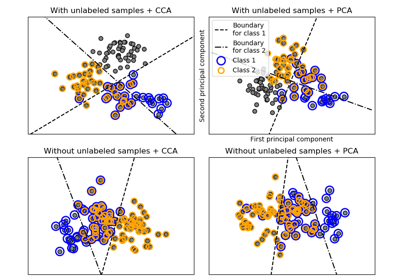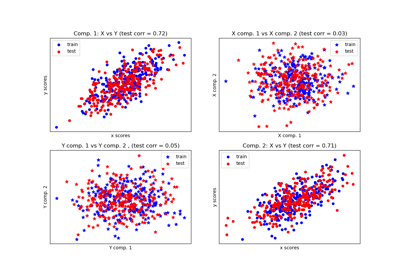/scikit-learn

# sklearn.cross_decomposition.CCA

`class sklearn.cross_decomposition.CCA(n_components=2, scale=True, max_iter=500, tol=1e-06, copy=True)` [source]

CCA Canonical Correlation Analysis.

CCA inherits from PLS with mode=”B” and deflation_mode=”canonical”.

Read more in the User Guide.

Parameters: `n_components : int, (default 2).` number of components to keep. `scale : boolean, (default True)` whether to scale the data? `max_iter : an integer, (default 500)` the maximum number of iterations of the NIPALS inner loop `tol : non-negative real, default 1e-06.` the tolerance used in the iterative algorithm `copy : boolean` Whether the deflation be done on a copy. Let the default value to True unless you don’t care about side effects `x_weights_ : array, [p, n_components]` X block weights vectors. `y_weights_ : array, [q, n_components]` Y block weights vectors. `x_loadings_ : array, [p, n_components]` X block loadings vectors. `y_loadings_ : array, [q, n_components]` Y block loadings vectors. `x_scores_ : array, [n_samples, n_components]` X scores. `y_scores_ : array, [n_samples, n_components]` Y scores. `x_rotations_ : array, [p, n_components]` X block to latents rotations. `y_rotations_ : array, [q, n_components]` Y block to latents rotations. `n_iter_ : array-like` Number of iterations of the NIPALS inner loop for each component.

#### Notes

For each component k, find the weights u, v that maximizes max corr(Xk u, Yk v), such that `|u| = |v| = 1`

Note that it maximizes only the correlations between the scores.

The residual matrix of X (Xk+1) block is obtained by the deflation on the current X score: x_score.

The residual matrix of Y (Yk+1) block is obtained by deflation on the current Y score.

#### References

Jacob A. Wegelin. A survey of Partial Least Squares (PLS) methods, with emphasis on the two-block case. Technical Report 371, Department of Statistics, University of Washington, Seattle, 2000.

In french but still a reference: Tenenhaus, M. (1998). La regression PLS: theorie et pratique. Paris: Editions Technic.

#### Examples

```>>> from sklearn.cross_decomposition import CCA
>>> X = [[0., 0., 1.], [1.,0.,0.], [2.,2.,2.], [3.,5.,4.]]
>>> Y = [[0.1, -0.2], [0.9, 1.1], [6.2, 5.9], [11.9, 12.3]]
>>> cca = CCA(n_components=1)
>>> cca.fit(X, Y)
...
CCA(copy=True, max_iter=500, n_components=1, scale=True, tol=1e-06)
>>> X_c, Y_c = cca.transform(X, Y)
```

#### Methods

 `fit`(X, Y) Fit model to data. `fit_transform`(X[, y]) Learn and apply the dimension reduction on the train data. `get_params`([deep]) Get parameters for this estimator. `predict`(X[, copy]) Apply the dimension reduction learned on the train data. `score`(X, y[, sample_weight]) Returns the coefficient of determination R^2 of the prediction. `set_params`(**params) Set the parameters of this estimator. `transform`(X[, Y, copy]) Apply the dimension reduction learned on the train data.
`__init__(n_components=2, scale=True, max_iter=500, tol=1e-06, copy=True)` [source]
`fit(X, Y)` [source]

Fit model to data.

Parameters: `X : array-like, shape = [n_samples, n_features]` Training vectors, where n_samples is the number of samples and n_features is the number of predictors. `Y : array-like, shape = [n_samples, n_targets]` Target vectors, where n_samples is the number of samples and n_targets is the number of response variables.
`fit_transform(X, y=None)` [source]

Learn and apply the dimension reduction on the train data.

Parameters: `X : array-like, shape = [n_samples, n_features]` Training vectors, where n_samples is the number of samples and n_features is the number of predictors. `y : array-like, shape = [n_samples, n_targets]` Target vectors, where n_samples is the number of samples and n_targets is the number of response variables. x_scores if Y is not given, (x_scores, y_scores) otherwise.
`get_params(deep=True)` [source]

Get parameters for this estimator.

Parameters: `deep : boolean, optional` If True, will return the parameters for this estimator and contained subobjects that are estimators. `params : mapping of string to any` Parameter names mapped to their values.
`predict(X, copy=True)` [source]

Apply the dimension reduction learned on the train data.

Parameters: `X : array-like, shape = [n_samples, n_features]` Training vectors, where n_samples is the number of samples and n_features is the number of predictors. `copy : boolean, default True` Whether to copy X and Y, or perform in-place normalization.

#### Notes

This call requires the estimation of a p x q matrix, which may be an issue in high dimensional space.

`score(X, y, sample_weight=None)` [source]

Returns the coefficient of determination R^2 of the prediction.

The coefficient R^2 is defined as (1 - u/v), where u is the residual sum of squares ((y_true - y_pred) ** 2).sum() and v is the total sum of squares ((y_true - y_true.mean()) ** 2).sum(). The best possible score is 1.0 and it can be negative (because the model can be arbitrarily worse). A constant model that always predicts the expected value of y, disregarding the input features, would get a R^2 score of 0.0.

Parameters: `X : array-like, shape = (n_samples, n_features)` Test samples. For some estimators this may be a precomputed kernel matrix instead, shape = (n_samples, n_samples_fitted], where n_samples_fitted is the number of samples used in the fitting for the estimator. `y : array-like, shape = (n_samples) or (n_samples, n_outputs)` True values for X. `sample_weight : array-like, shape = [n_samples], optional` Sample weights. `score : float` R^2 of self.predict(X) wrt. y.
`set_params(**params)` [source]

Set the parameters of this estimator.

The method works on simple estimators as well as on nested objects (such as pipelines). The latter have parameters of the form `<component>__<parameter>` so that it’s possible to update each component of a nested object.

Returns: self
`transform(X, Y=None, copy=True)` [source]

Apply the dimension reduction learned on the train data.

Parameters: `X : array-like, shape = [n_samples, n_features]` Training vectors, where n_samples is the number of samples and n_features is the number of predictors. `Y : array-like, shape = [n_samples, n_targets]` Target vectors, where n_samples is the number of samples and n_targets is the number of response variables. `copy : boolean, default True` Whether to copy X and Y, or perform in-place normalization. x_scores if Y is not given, (x_scores, y_scores) otherwise.

## Examples using `sklearn.cross_decomposition.CCA`Multilabel classificationCompare cross decomposition methods